# Arrays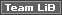Your first question is probably, "What is an array?" Earlier, we said that variables are just temporary storage. If you think of a variable as a can of cola, then you can think of an array as a six-pack of cola. They are collections of variables , all with the same name and the same data type. Elements in an array are identified by their index - a number indicating their position in the array.

Arrays are used for collecting together a number of similar variables. Variables themselves are useful for holding specific information about a certain object, property, or value. For example, if you want to store a number suggested by the user , you can create a variable to hold that value and then assign the value to the variable. These are the two lines of code that you might use to do that:

`   Dim intNum As Integer     intNum = InputBox("Please enter guess number 1", "Guess!")   `

The first line of code declares the variable as an integer. That is to say that the variable intNum will be able to hold any whole number between -32,768 and +32,767.

The second line of code assigns to the variable a number that has to be entered by the user. If you wanted the user to enter two numbers and you wanted to store the two values concurrently, you could create two variables as shown in the code below:

`   'Declare variables     Dim iNum As Integer     Dim iNum2 As Integer     'Assign values to variables     iNum = InputBox("Please enter guess number 1", "Guess!")     iNum2 = InputBox("Please enter guess number 2", "Guess!")   `

What if you want the user to be able to make five guesses... or twenty... or more? The answer is that your code could become very lengthy and repetitive. Given that the potential number of bugs in any program usually increases in proportion to the number of lines of code, you probably won't want to use the method shown above. What you need is a method for storing a collection of related variables together. That's just what an array provides.

The following are examples of items of data that you may want to collect in an array:

• The values of ten guesses made by the user

• Each individual letter making up a single string

• The enabled property of a number of different command buttons on a form

• The values of all the controls on a specific form

To show this at work, let's rewrite the code above to allow the user to make 10 initial guesses:

### Try It Out-Declaring and Populating an Array

1. Open IceCream.mdb if it's not open already, and select the Modules tab from the database window. Open your module for the chapter and enter the following code:

`   Public Sub ArrayExample()     Dim i As Integer     Dim intNum(1 To 10) As Integer     For i = 1 To 10     intNum(i) = InputBox("Please enter guess " & i, "Guess!")     Next i     For i = 1 To 10     Debug.Print "Guess number " & i & " = " & intNum(i)     Next i     End Sub   `
2. Run the ArrayExample procedure by typing ArrayExample in the Immediate window and hitting the Enter key. You'll be prompted to enter ten integers. Once you have entered the last of the ten integers, all ten will be displayed.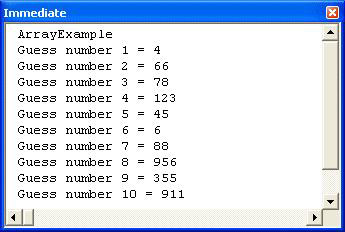How It Works

Declaring an array is easy. We simply place parentheses indicating the array's dimensions after its name. So whereas this:

` Dim intNum As Integer `

declares an Integer type variable called intNum, this:

` Dim intNum (1 To 10) As Integer `

declares an array of ten Integer type variables called intNum . This tells VBA that intNum is to hold ten separate values, each of which will be a whole number between -32,768 and 32,767.

Note that an array need not hold Integer type variables. An array can hold any of the following data types:

• Integer

• Long

• Single

• Double

• Variant

• Currency

• String

• Boolean

• Byte

• Date

• Object

• User Defined Types

However, all elements of the array must be of the same data type. In other words, one array will not be able to store both strings and integers (although it could store Variant type variables with differing subtypes ).

` For i = 1 To 10   intNum(i) = InputBox("Please enter guess" & i, "Guess!") Next i `

Now all that's needed is to populate the ten elements of the array with guesses from the user. Individual elements of the array are identified by their index (the number which appears in parentheses after the variable's name) so we create a simple For...Next loop and use the loop counter - in this case, the variable i - to refer to the elements of the array.

The ten elements of the intNum array are referred to as intNum(1) , intNum(2) , intNum(3) , ... intNum(10) .

` intNum(i) = InputBox("Please enter guess " & i, "Guess!") `

To make this rather tedious task easier on the user, we have also used the loop counter, i , to indicate to the user how many guesses they have had:

` For i = 1 To 10   Debug.Print "Guess number " & i & " = " & intNum(i) Next i `

Having stored the results of all the guesses in an array, we then loop through the elements of the array to display the results in the debug window.

Note that if we wanted to allow the user to make twenty guesses instead of ten, we need only alter three lines of code. In fact, we could reduce this to one line by replacing the value 10 in the code above with the constant ciNumberOfGuesses . The procedure would then look like this:

`   Sub ArrayExampleWithConstant()     Const ciNumberOfGuesses = 10     Dim i As Integer     Dim intNum(1 To ciNumberOfGuesses) As Integer     For i = 1 To ciNumberOfGuesses     intNum(i) = InputBox("Please enter guess " & i, "Guess!")     Next i     For i = 1 To ciNumberOfGuesses     Debug.Print "Guess number " & i & " = " & intNum(i)     Next i     End Sub   `

To change the number of guesses that the user is allowed, we now need only to change the value of ciNumberOfGuesses .

### Static Arrays

The examples above all made use of static arrays . That is to say, the number of elements in the array was fixed when the array was first declared. When you declare an array in this manner, the number of elements can't be changed.

Don't confuse static arrays with static variables. A static variable is one which has been declared with the Static statement and preserves its values between calls. A static array is one whose dimensions are fixed when it is declared.

Static (that is, fixed-dimension) arrays can be declared with any of the following statements Dim , Static , Private , or Public .

For example, typing the following in the Declarations section of a form's code module would declare an array with a fixed number of elements which would be visible to all procedures in that form:

`   Option Compare Database     Option Explicit     Private intNum(1 To 10) As Integer   `

Whereas the following, if typed in the Declarations section of a standard code module, would declare an array with a fixed number of elements which was visible to all procedures throughout all code modules, forms, and reports .

`   Option Compare Database     Option Explicit     Public intNum(1 To 10) As Integer   `

After you have created a static array, the elements of the array are initialized. All that means is that VBA gives these elements default values. The values with which they are initialized depend on the data type of the elements.

Data type

Initialization value

Any numeric

String (variable length)

Zero-length string ( "" )

String (fixed length)

A fixed-length string of Chr\$(0) characters

Variant

Empty

#### Upper and Lower Bounds

The bounds of an array are its lowest and highest indexes. The lower bound of an array can be set to any integer value (but we usually set it to ). Had we wanted to, we could have typed:

`   Dim intNum(23 to 32) As Integer   `

This would also have given us an array which could hold a maximum of ten integers, but whose index would run from 23 to 32 . To populate this array, we could use the following For ... Next loop:

`   For i = 23 To 32     intNum(i) = ...     Next i   `

Alternatively, if we had wished, we could have omitted the lower bound and typed instead:

`   Dim intNum(10) As Integer   `

However, you should note that, if you do not explicitly specify a lower bound, Access will use as the lower bound. In other words, the line of code above is evaluated as:

`   Dim intNum(0 to 10) As Integer   `

This means that the array intNum() will be able to hold eleven values.

If you want Access to use 1 instead of as the default lower bound for arrays, you should include the following line of code in the Declarations section of the code module:

`   Option Base 1   `

Just so you know, you can no longer change the option base to anything other than in the newer VB.NET. For this reason, it's probably not good practice in VBA.

### Dynamic Arrays

You may not know at the outset how many elements are required in your array. In this case, you should declare a dynamic array. You do this by placing empty parentheses after the array name you can still use the Dim , Static , Private , or Public keywords. The ReDim statement is then used later in the procedure to dynamically set the lower and upper bounds of the array.

For example, we could modify our original procedure to allow the user to specify how many guesses they want:

### Try It Out-Dynamic Arrays

1. Create a new procedure called DynamicArrayExample with the following code:

`   Sub DynamicArrayExample()     Dim i As Integer     Dim intGuessCount As Integer     Dim intNum() As Integer     intGuessCount = InputBox("How many guesses do you want?")     ReDim intNum(1 To intGuessCount)     For i = 1 To intGuessCount     intNum(i) = InputBox("Please enter guess " & i, _     "Guess!")     Next i     For i = 1 To intGuessCount     Debug.Print "Guess number " & i & " = " & intNum(i)     Next i     End Sub   `
2. Switch to the Immediate window and run the procedure.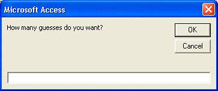First, you're asked how many guesses you want.

3. Enter the number of tries you are going to have and press the OK button.

4. For each new dialog enter any integer (within reason!).

5. When you're finished you'll see the results in the Immediate window: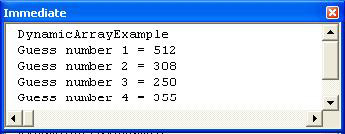How It Works

You've seen some of the code before, so we'll just look at the new stuff here. The first thing to notice is that in the Dim statement we don't specify how many elements the array will contain:

`   Dim intNum() As Integer   `

The next thing to do is ask the user how many guesses they want - this will determine the number of array elements:

`   intGuessCount = InputBox("How many guesses do you want?")   `

Now we have the number of elements we can use ReDim to specify the size of the array:

`   ReDim intNum(1 To intGuessCount)   `

And finally, we use the number of guesses as the maximum in the loop.

`   For i = 1 To intGuessCount   `

This technique saves having to declare a large array just because you aren't sure how many elements it's going to have. In fact you can take this one step further, and increase or decrease the size of the array as you go along, and here, you only use as much memory as you need.

### Try It Out-Very Dynamic Arrays

1. Create another procedure called VeryDynamicArray , like so:

`   Public Sub VeryDynamicArray()     Dim i As Integer     Dim intGuess As Integer     Dim intNum() As Integer     ReDim intNum(0)     i = 1     Do     intGuess = InputBox("Please enter guess " & i & _     vbCr & "Use -1 to exit", "Guess!")     If intGuess <> -1 Then     ReDim Preserve intNum(i)     intNum(i) = intGuess     i = i + 1     End If     Loop Until intGuess = -1     For i = 1 To UBound(intNum)     Debug.Print "Guess number " & i & " = " & intNum(i)     Next i     End Sub   `
2. Switch to the Immediate window and run this code by typing in VeryDynamicArray followed by the Enter key.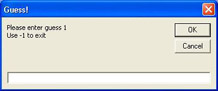Notice that you haven't been asked how many guesses you want. You can just type in as many guesses as you like, and when you are finished you should enter -1 .

3. Now switch back to the Immediate window to see the results: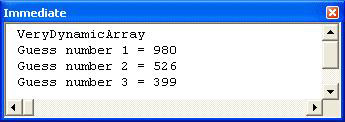How It Works

This is quite different from the previous two examples, so we'll look at this in more detail.

The first thing is defining the variables:

`   Dim i As Integer     Dim intGuess   As Integer     Dim intNum()   As Integer   `

Then we ReDim the array to make sure it's got at least one element in it and set the initial count number. We'll need these later.

`   ReDim intNum(0)     i = 1   `

Now we can start our loop. Notice that we are using a Do loop, so we can decide at run time when to end the loop.

`   Do   `

Now we are inside the loop so we ask for our guess. We've used a constant, vbCr ( carriage return), to force the string to be split onto two lines. This just makes it easier for the user to read.

`   intGuess = InputBox("Please enter guess " & i & _     vbCr & "Use -1 to exit", "Guess!")   `

Now we check to see if the user has entered -1 , as we only want to add the number to the array if they haven't:

`   If intGuess <> -1 Then   `

If they have entered a number other than -1, we do three things. First, we increase the size of the array. Notice the use of Preserve to make sure that any existing array elements are kept (we'll look at this a little later). Then we assign the new array element to the user guess, and then we increase the count number, before ending the If statement.

`   ReDim Preserve intNum(i)     intNum(i) = intGuess     i = i + 1     End If   `

Now we come to the end of the loop, and we only stop the loop if the user has entered -1 .

`   Loop Until intGuess = -1   `

Now we need to print out the guesses. To find out how many times we should loop, we use UBound to find out the upper bound of the array.

`   For i = 1 To UBound(intNum)     Debug.Print "Guess number " & i & " = " & intNum(i)     Next i   `

So, you can see that arrays are quite flexible, and you don't need to know in advance how many elements are required.

#### Redimensioning Existing Arrays

The ReDim statement can also be used to change the size of a dynamic array that already has a known number of elements set by a previous ReDim statement. For example, if you have an array of ten elements, declared with the following code:

`   Dim iNum() As Integer     ...     ReDim iNum(1 To 10)   `

You can reduce the number of elements in the array later in the procedure to four, with the single line of code:

`   ReDim iNum(1 To 4)   `

You might want to do this if you had a large array but now only need a small one. Reducing the size of the array will save memory.

Normally, when you change the size of an array, you lose all the values that were in that array - it is reinitialized. However, you can avoid this by using the Preserve keyword. The following line of code would have the same effect as the previous one, except that the values stored in iNum(1) , iNum(2) , iNum(3) , and iNum(4) would remain unchanged.

`   ReDim Preserve iNum(1 To 4)   `

#### The Dangers of ReDim

There's one important point to notice when using ReDim , which can be shown with a couple of lines of code:

`   Dim intNum() As Integer     ReDim intNun(10)   `

Notice that the variable in the second statement is different from that in the first. This can happen quite easily as a typing mistake. So what happens here? Usually when you use a variable that hasn't been declared, VBA gives you an error - but not with ReDim . This is because the ReDim command acts like a declaration if you don't use Option Explicit . In the above example this leads to two arrays, which can cause errors in your code. This actually happened to us while we were creating one of the above examples.

If you are getting errors telling you that an index is out of bounds, but you are sure you have dimensioned it correctly, then check your spelling - you might have given the wrong name to the variable in your ReDim statement. Using Option Explicit in all of your code modules will eliminate this problem.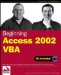Beginning Access 2002 VBA (Programmer to Programmer)
ISBN: 0764544020
EAN: 2147483647
Year: 2003
Pages: 256